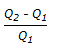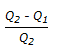# Chemical Engineering - Fluid Mechanics

1.

The continuity equation of fluid mechanics utilises the principle of conservation of

 A. momentum B. mass C. energy D. both (b) & (c)

Explanation:

No answer description available for this question. Let us discuss.

2.

Stoke's law is valid, when the particle Reynolds number is

 A. < 1 B. > 1 C. < 5 D. none of these

Explanation:

No answer description available for this question. Let us discuss.

3.

Which of the following is not a dimension-less parameter ?

 A. Pressure-co-efficient B. Froude number C. Kinematic viscosity D. Weber number

Explanation:

No answer description available for this question. Let us discuss.

4.

In which of the following cases, it is possible for flow to occur from low pressure to high pressure ?

 A. Flow of liquid upward in a vertical pipe. B. Flow through a converging section. C. Flow of air downward in a pipe. D. Impossible in a constant cross-section conduit.

Explanation:

No answer description available for this question. Let us discuss.

5.

The percentage slip in a reciprocating pump set is given by the % of (where, Q1 = actual discharge Q2 = theoretical discharge)

 A. Q1/Q2 B. Q2/Q1 C.D.